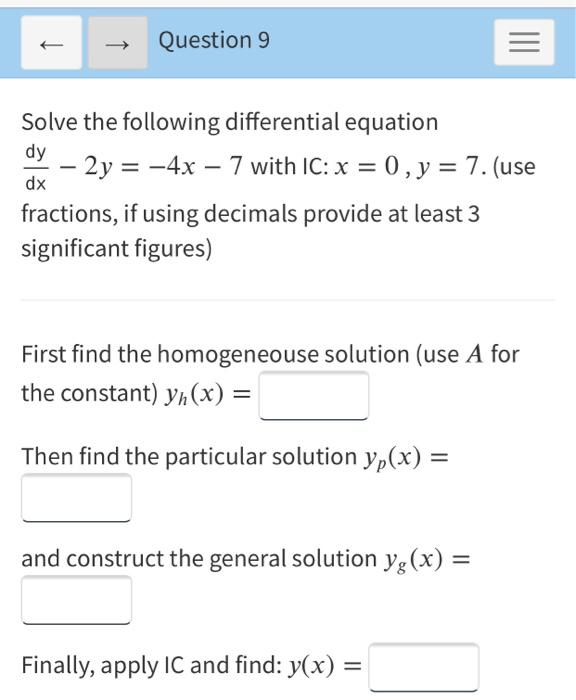### Create an Account

Already have account?

### Forgot Your Password ?

Home / Questions / Question 9 III Solve the following differential equation dy – 2y = -4x – 7 with IC: x = 0,...

# Question 9 III Solve the following differential equation dy – 2y = -4x – 7 with IC: x = 0, y = 7. (use fractions, if using decimals provide at least 3 significant figures) dx First find the homogeneou

Question 9 III Solve the following differential equation dy – 2y = -4x – 7 with IC: x = 0, y = 7. (use fractions, if using decimals provide at least 3 significant figures) dx First find the homogeneouse solution (use A for the constant) yn(x) = Then find the particular solution yp(x) = and construct the general solution yg(x) = Finally, apply IC and find: y(x) =Apr 15 2021 View more View Less

#### Answer (Solved)Subscribe To Get Solution